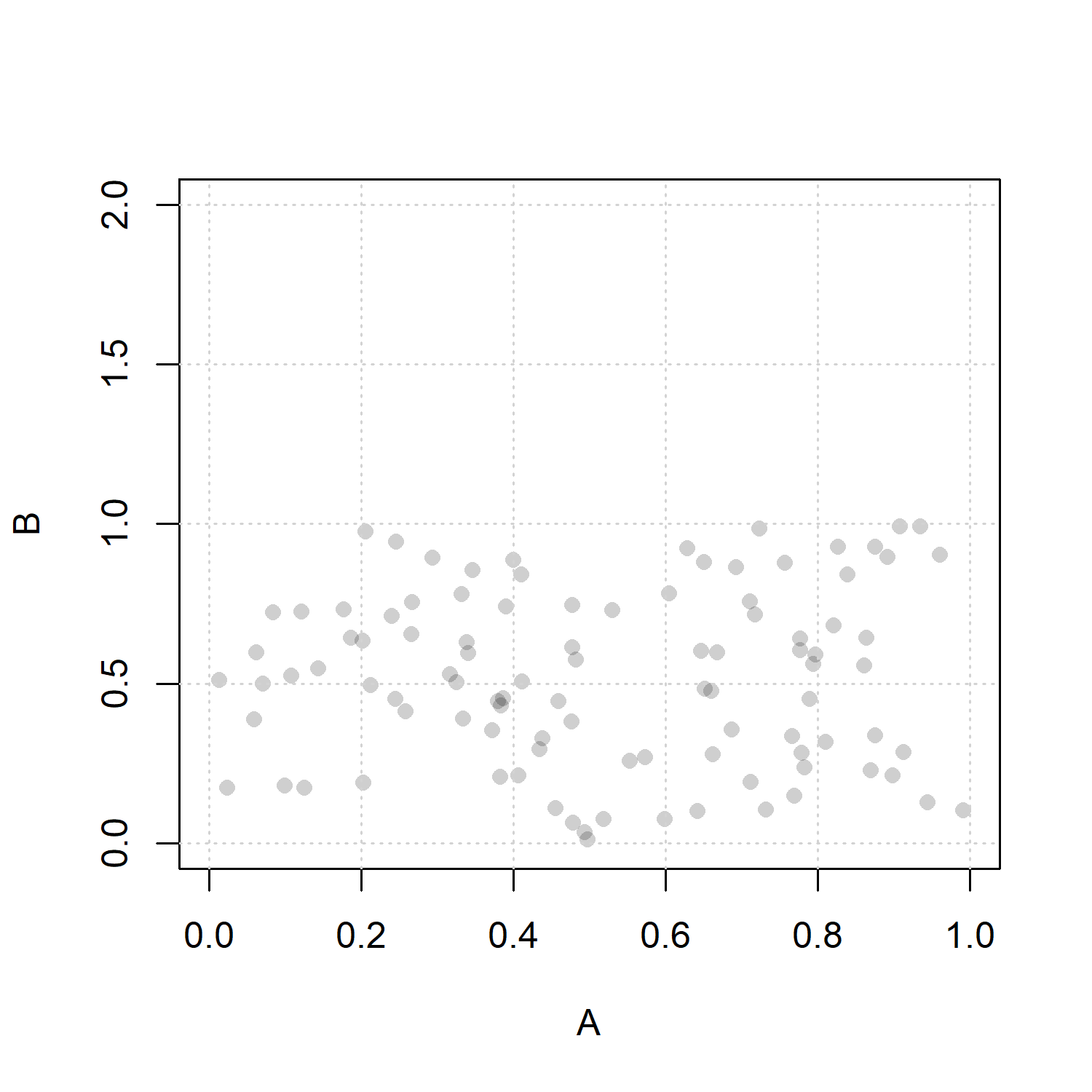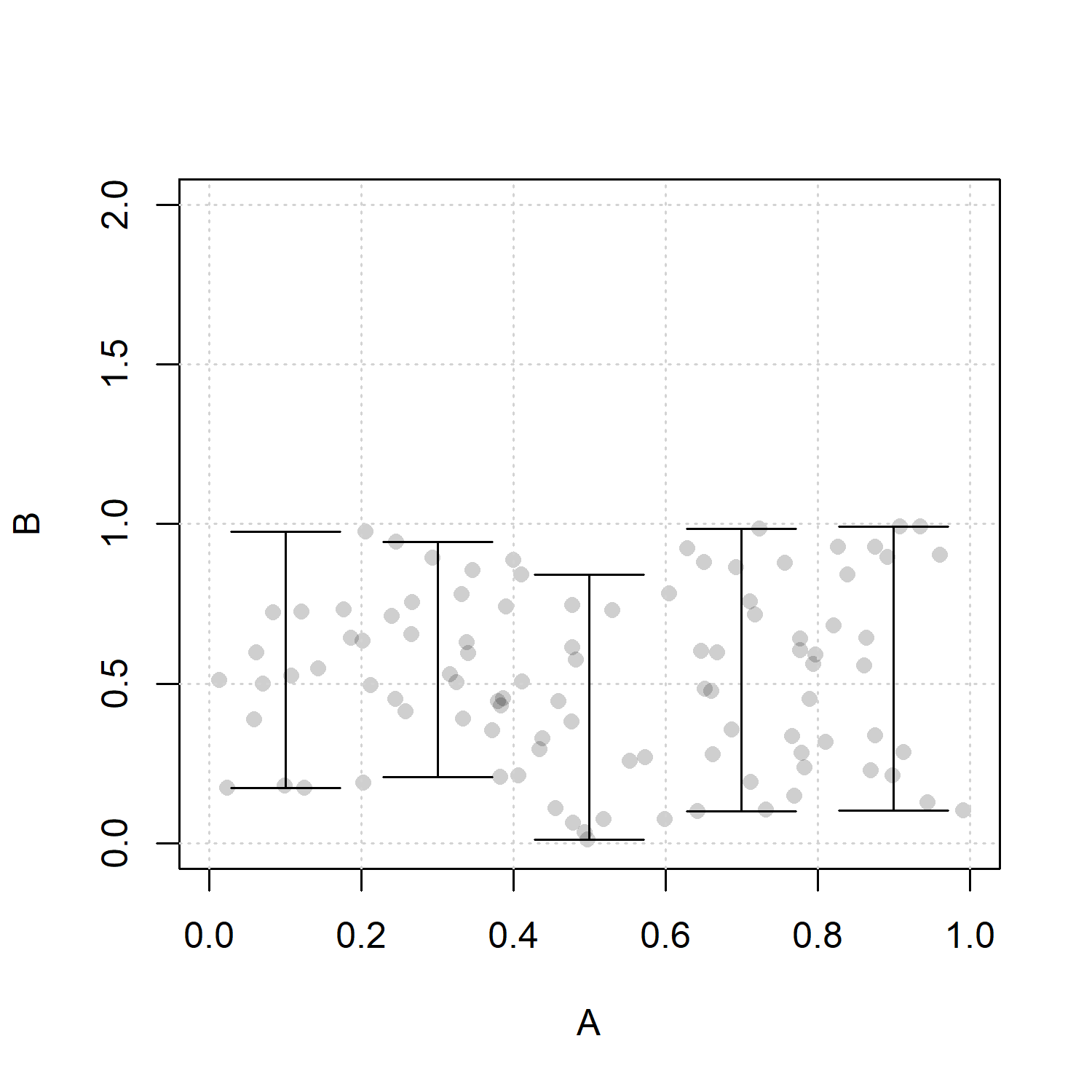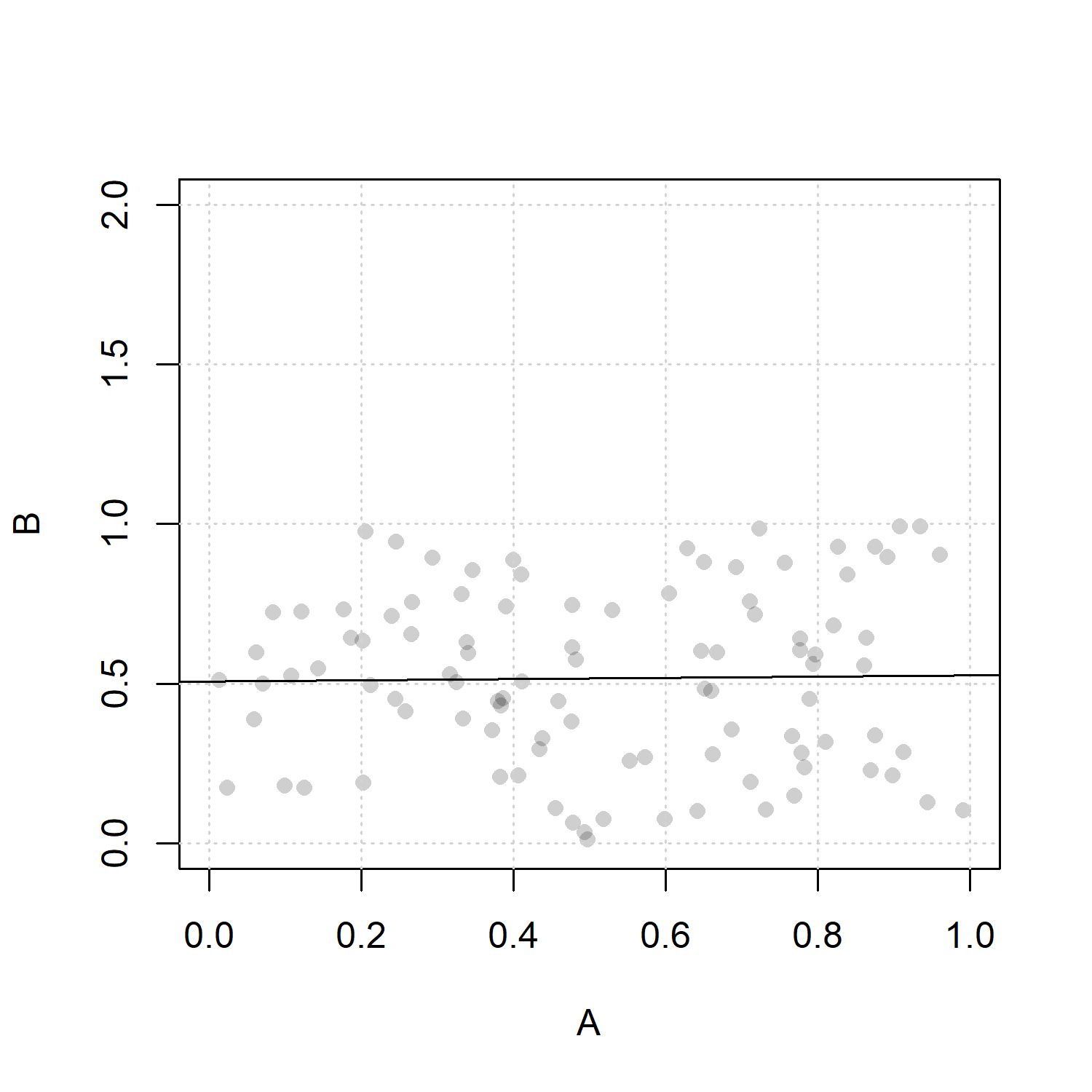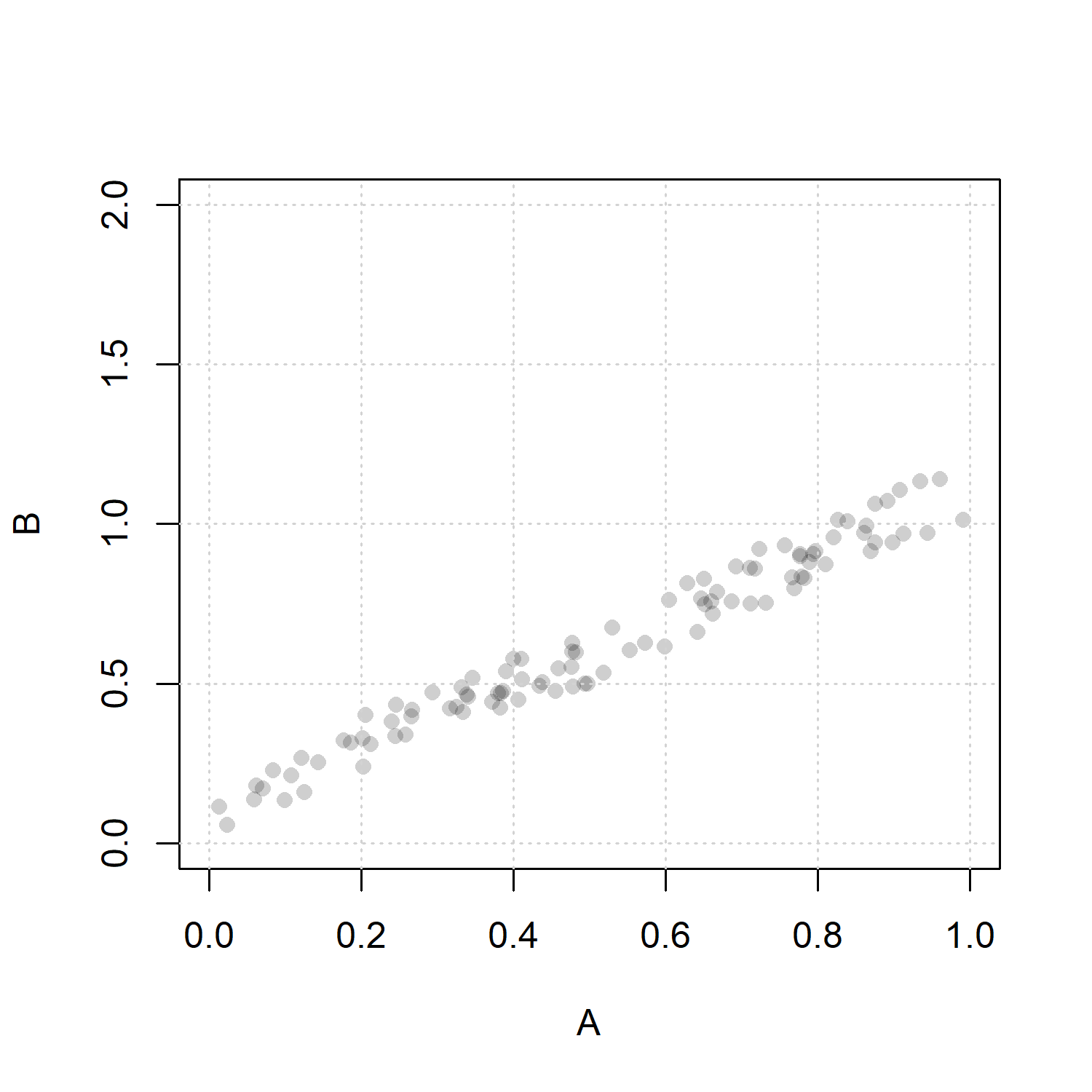# Predictive modeling in linguistics

Published

15 Jul 2023 12-34-56

# 1 Fundamentals of regression modeling, part 1

In some sense, this whole course will be on the notion of correlation, which is why I want to spend some time on reminding us all on what that is/means.

Definition: Two variables `A` and `B` are correlated

• if knowing the value/range of `A` makes it easier to ‘predict’ (better) the value/range of `B` than if one doesn’t know the value/range of `A`;
• if knowing the value/range of `B` makes it easier to ‘predict’ (better) the value/range of `A` than if one doesn’t know the value/range of `B`.

Here is an example where knowing `A` (or `B`) does not help ‘predicting’ `B` (or `A`):``````
Pearson's product-moment correlation

data:  A and B
t = 0.16863, df = 98, p-value = 0.8664
alternative hypothesis: true correlation is not equal to 0
95 percent confidence interval:
-0.1799881  0.2127386
sample estimates:
cor
0.01703215 ``````

Why does knowing `A` (or `B`) not help ‘predicting’ `B` (or `A`)? Because, for instance, no matter which value range of `A` you pick, you can’t predict `B` very well (and vice versa):And we can exemplify that easily with a regression line as well:By contrast, here is an example where knowing `A` (or `B`) does help ‘predicting’ `B` (or `A`):``````
Pearson's product-moment correlation

data:  A and B
t = 48.903, df = 98, p-value < 2.2e-16
alternative hypothesis: true correlation is not equal to 0
95 percent confidence interval:
0.9705438 0.9866034
sample estimates:
cor
0.9801194 ``````

Why does knowing `A` (or `B`) help ‘predicting’ `B` (or `A`)? Because, for instance, knowing the value range of `A` makes you predict `B` better (and vice versa):